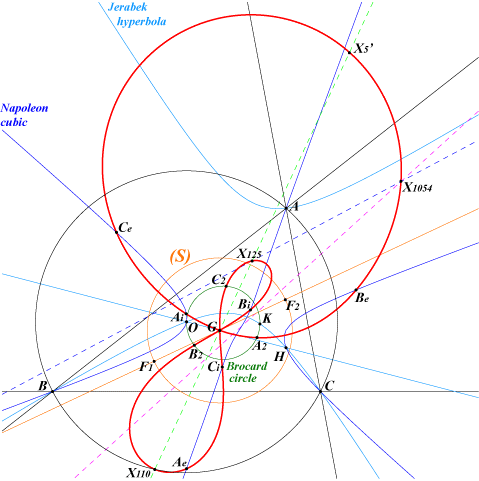too complicated to be written here. Click on the link to download a text file.X(2), X(110), X(125), X(1054), X(3448) Ae, Be, Ce : vertices of the outer Napoleon triangle Ai, Bi, Ci : vertices of the inner Napoleon triangle A2, B2, C2 : vertices of the second Brocard triangle extraversions of X(1054) more generally, Psi-transform (see below) of any point on the Napoleon cubic.An orthopivotal pK has its orthopivot on the Napoleon cubic. Its singular focus lies on Q041. Q041 is a tricircular sextic passing through G which is a triple point. It is the transform of the Napoleon cubic under Psi described as follows. Denote by F1, F2 the foci of the Steiner inellipse. Psi(P) is the reflection in the line F1F2 of the inverse of P in the circle (S) with diameter F1F2. Psi is clearly an involution which swaps : the circumcircle and the Brocard circle. In particular A, B, C are transformed into A2, B2, C2 (vertices of the second Brocard triangle). any line through G and its reflection in F1F2. In particular, the Euler line is transformed into the line G-X(110) and the points X(3), X(4), X(5) into X(110), X(125), X(3448) respectively. the perpendicular bisector of BC and a circle through G, X(110), Ae, Ai. Note that Psi swaps Ae and Ai. the altitude AH and a circle through G and X(125). the Brocard axis OK and the Parry circle. See the analogous Q048, Q119.